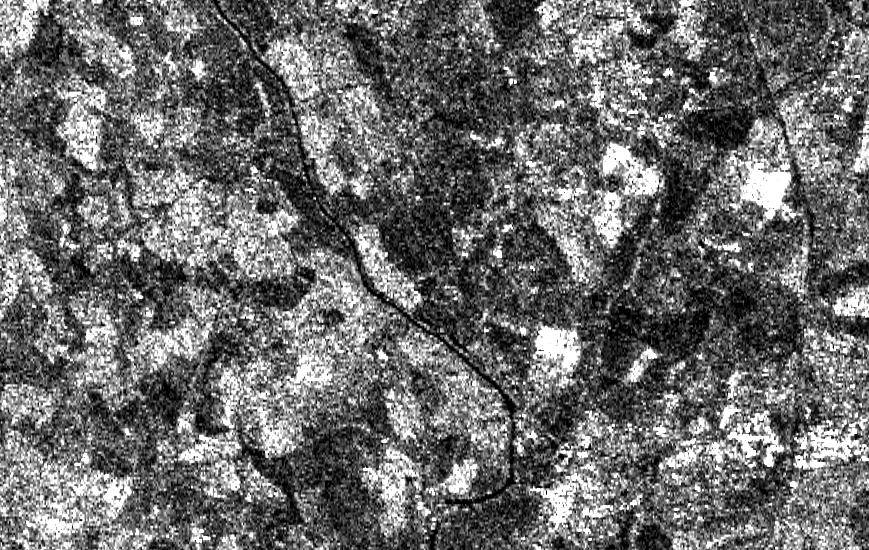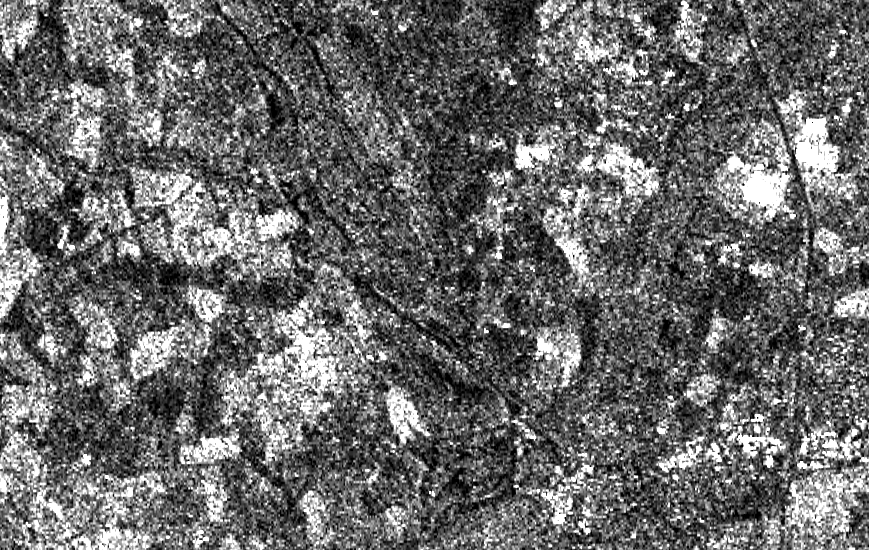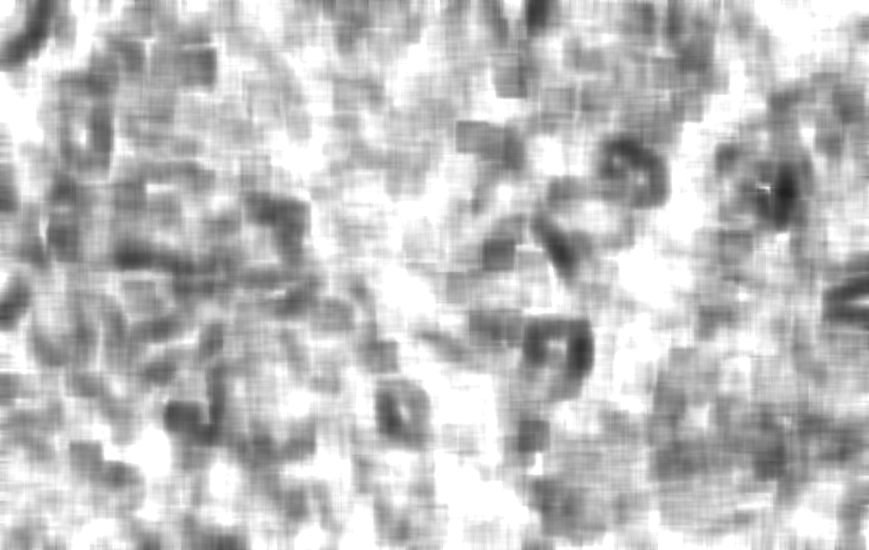# CorrelChDet.cxx¶

This example illustrates the class otb::CorrelationChangeDetector for detecting changes between pairs of images. This filter computes the correlation coefficient in the neighborhood of each pixel of the pair of images to be compared.ERS Images for Change Detection. Left: Before the flood. Middle: during the flood. Right: result of the correlation change detector

Example usage:

```./CorrelChDet Input/ERSBefore.png Input/ERSAfter.png Output/CorrChDet.tif 15
```

Example source code (CorrelChDet.cxx):

```#include "otbImageFileReader.h"
#include "otbImageFileWriter.h"
#include "otbImage.h"
#include "itkShiftScaleImageFilter.h"
#include "otbCommandProgressUpdate.h"
#include "otbCorrelationChangeDetector.h"

int main(int argc, char* argv[])
{

if (argc < 5)
{
std::cerr << "Usage: " << std::endl;
std::cerr << argv << " inputImageFile1 inputImageFile2 "
return -1;
}

// Define the dimension of the images
const unsigned int Dimension = 2;

// We start by declaring the types for the two input images, the
// change image and the image to be stored in a file for visualization.
using InternalPixelType = float;
using OutputPixelType   = unsigned char;
using InputImageType1   = otb::Image<InternalPixelType, Dimension>;
using InputImageType2   = otb::Image<InternalPixelType, Dimension>;
using ChangeImageType   = otb::Image<InternalPixelType, Dimension>;
using OutputImageType   = otb::Image<OutputPixelType, Dimension>;

// We can now declare the types for the readers. Since the images
// can be very large, we will force the pipeline to use
// streaming. For this purpose, the file writer will be
// streamed.
using WriterType  = otb::ImageFileWriter<OutputImageType>;

// The change detector will give a response which is normalized
// between 0 and 1.
// Before saving the image to a file in, for instance, PNG format, we will
// rescale the results of the change detection in order to use all
// the output pixel type range of values.

// The CorrelationChangeDetector is templated over
// the types of the two input images and the type of the generated change
// image.
using FilterType = otb::CorrelationChangeDetector<InputImageType1, InputImageType2, ChangeImageType>;

// The different elements of the pipeline can now be instantiated.
WriterType::Pointer   writer         = WriterType::New();
FilterType::Pointer   filter         = FilterType::New();
const char*           inputFilename1 = argv;
const char*           inputFilename2 = argv;
const char*           outputFilename = argv;

// We set the parameters of the different elements of the pipeline.
writer->SetFileName(outputFilename);

float scale = itk::NumericTraits<OutputPixelType>::max();
rescaler->SetScale(scale);

// The only parameter for this change detector is the radius of
// the window used for computing the correlation coefficient.

// We build the pipeline by plugging all the elements together.
rescaler->SetInput(filter->GetOutput());
writer->SetInput(rescaler->GetOutput());

// Since the processing time of large images can be long, it is
// interesting to monitor the evolution of the computation. In
// order to do so, the change detectors can use the
// command/observer design pattern. This is easily done by
// attaching an observer to the filter.
using CommandType = otb::CommandProgressUpdate<FilterType>;

CommandType::Pointer observer = CommandType::New();